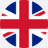# CBSE CGPA to Percentage Calculator: Conversion Formula and Calculator

The CBSE (Central Board of Secondary Education) is one of the most popular boards in India for secondary and senior secondary education. CBSE students in 10th grade are graded on a 10-point scale, known as CGPA (Cumulative Grade Point Average), which indicates their overall academic performance. It is important to note that the CGPA grading system is applicable only for CBSE classes IX and X.

Also read: How to Convert CGPA to Percentage?

## CBSE CGPA to Percentage Conversion Formula

When students apply for higher education after school, many universities and colleges require them to submit their percentage scores rather than their CGPA. Therefore, it is essential for CBSE students to convert their CGPA to a percentage score accurately.

The process of converting CGPA to percentage is relatively straightforward. The CBSE has provided a formula for calculating the percentage from CGPA.

### How to convert CBSE CGPA into percentage?

To convert CBSE CGPA to a percentage, simply multiply your CGPA by 9.5.

Percentage = CGPA x 9.5

This formula is available on the CBSE's official website for students in grades IX and X. For example, if you had a 9.4 cumulative grade point average (CGPA), the comparable percentage would be 9.4*9.5 = 89.3%.

Let us understand this with an another example:

Suppose a student has scored a CGPA of 8.2. We will use the above-mentioned formula to convert this CGPA to a percentage.

Percentage = 8.2 x 9.5

Percentage = 77.9%

Therefore, the student percentage is 77.9%.

It is important to note that the CGPA scale ranges from 0 to 10, while the percentage scale ranges from 0 to 100. Thus, multiplying the CGPA by 9.5 helps convert it to a percentage out of 100.

## CBSE CGPA to Percentage Calculator

To use a CGPA to percentage calculator, students need to enter their CGPA score into the calculator, and the tool will automatically provide their percentage score. Students can use these calculators to quickly and accurately convert their CGPA to percentages.

In conclusion, CGPA helps assess a student's overall academic performance, but more is needed for further education applications. Therefore, students must accurately convert their CGPA to a percentage using the correct formula or an online CGPA to percentage calculator to ensure they meet the requirements of their chosen institution.

### How do i make a cbse percentage out of a CGPA?

To convert your CBSE CGPA to a percentage, just multiply your CGPA by 9.5. This formula is available for grades IX and X on the CBSE's official website. For instance, if your CGPA is 9.4, the comparable percentage is 9.4*9.5 = 89.3 percent.

The percentage may be computed by dividing the value by the entire value and multiplying the result by 100. (value/total value) 100 percent is the formula for calculating percentages.

To convert your CGPA to a percentage, just multiply your CGPA by 9.5. This formula is available for grades IX and X on the CBSE's official website. For instance, if your CGPA is 9.4, the comparable percentage is 9.4*9.5 = 89.3%.

How to convert the cbse percentage to CGPA?

To convert a CBSE percentage to a CGPA (out of ten), divide it by 9.5, and you'll get your CGPA. For instance, to convert 75% to CGPA, divide it by 9.5; the result is 7.9, which is the CGPA out of ten.

To convert your CGPA to a percentage, just multiply your CGPA by 9.5. This formula is available for grades IX and X on the CBSE's official site. For instance, if your CGPA is 9.4, the comparable percentage is 9.4*9.5 = 89.3%.

### What is the 10 CGPA to percentage?

10 CGPA in percentage = 10 x 9.5 = 95%

### What is the 8.2 CGPA in percentage?

8.2 CGPA in percentage = 8.2 x 9.5 = 77.9%

### What is the 9.4 CGPA in percentage?

9.4 CGPA in percentage = 9.4 x 9.5 = 90.3%

### What is the 10 CGPA in percentage?

10 CGPA in percentage = 10 x 9.5 = 95%

### What is the 9.5 CGPA in percentage?

9.5 CGPA in percentage = 9.5 x 9.5 = 90.25%

Related pages,

## FAQ’s:

### What is CGPA?

CGPA stands for Cumulative Grade Point Average. CBSE uses a 10-point grading system to evaluate students overall academic performance for classes IX and X.

### What is the formula to convert CGPA to percentage?

The formula to convert CGPA to percentage is Percentage = CGPA x 9.5. For example, if a student has a CGPA of 8.2, their percentage score would be 8.2 x 9.5 = 77.9%.

### Why do colleges and universities require a percentage score instead of CGPA?

Most colleges and universities require a percentage score instead of CGPA because they use it as a standard measure to compare a student’s academic performance. Additionally, some institutions may have a specific minimum percentage requirement for admission or scholarships.

### Can CGPA be converted directly to a percentage without using the formula?

No, CGPA cannot be converted directly to a percentage as the scales differ. Therefore, the formula Percentage = CGPA x 9.5 must be used to calculate the percentage score.

### Are CGPA and percentage scores equivalent?

No, CGPA and percentage scores are not equivalent. CGPA is a scale ranging from 0 to 10, while the percentage scale ranges from 0 to 100. CGPA is a relative grading system, while the percentage is an absolute grading system.

### Is there any difference in the conversion formula for different education boards?

Yes, different education boards may have slightly different conversion formulas. Therefore, students must use the specific conversion formula their education board provides.

### Is using an online calculator for CGPA to percentage conversion accurate?

Yes, using an online calculator for CGPA to percentage conversion can be accurate if the calculator uses the correct formula. However, students should double-check the results of an online calculator to ensure accuracy.£
GBP